Chapter 12. Acids and Bases

# Autoionization of Water

Learning Objectives

1. Describe the autoionization of water.
2. Calculate the concentrations of H+ and OH in solutions, knowing the other concentration.

We have already seen that H2O can act as an acid or a base: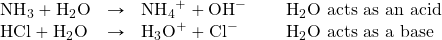It may not surprise you to learn, then, that within any given sample of water, some H2O molecules are acting as acids, and other H2O molecules are acting as bases. The chemical equation is as follows: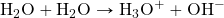This occurs only to a very small degree: only about 6 in 108 H2O molecules are participating in this process, which is called the autoionization of water. At this level, the concentration of both H+(aq) and OH(aq) in a sample of pure H2O is about 1.0 × 10−7 M. If we use square brackets around a dissolved species to imply the molar concentration of that species, we have: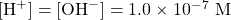for any sample of pure water, because H2O can act as both an acid and a base. The product of these two concentrations is 1.0 × 10−14: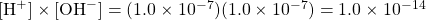In acids, the concentration of H+(aq) — written as [H+] — is greater than 1.0 × 10−7 M, while for bases the concentration of OH(aq) — [OH] — is greater than 1.0 × 10−7 M. However, the product of the two concentrations — [H+][OH] — is always equal to 1.0 × 10−14, no matter whether the aqueous solution is an acid, a base, or neutral: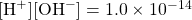This value of the product of concentrations is so important for aqueous solutions that it is called the autoionization constant of water and is denoted Kw:This means that if you know [H+] for a solution, you can calculate what [OH] has to be for the product to equal 1.0 × 10−14, or if you know [OH], you can calculate [H+]. This also implies that as one concentration goes up, the other must go down to compensate so that their product always equals the value of Kw.

Example 12.9

What is [OH] of an aqueous solution if [H+] is 1.0 × 10−4 M?

Solution
Using the expression and known value for Kw: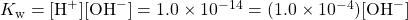We solve by dividing both sides of the equation by 1.0 × 10−4: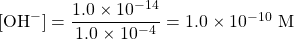It is assumed that the concentration unit is molarity, so [OH] is 1.0 × 10−10 M.

Test Yourself
What is [H+] of an aqueous solution if [OH] is 1.0 × 10−9 M?

1.0 × 10−5 M

When you have a solution of a particular acid or base, you need to look at the formula of the acid or base to determine the number of H+ or OH ions in the formula unit because [H+] or [OH] may not be the same as the concentration of the acid or base itself.

Example 12.10

What is [H+] in a 0.0044 M solution of Ca(OH)2?

Solution
We begin by determining [OH].

The concentration of the solute is 0.0044 M, but because Ca(OH)2 is a strong base, there are two OH ions in solution for every formula unit dissolved, so the actual [OH] is two times this, or 2 × 0.0044 M = 0.0088 M.

Now we can use the Kw expression: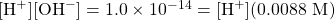Dividing both sides by 0.0088: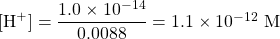[H+] has decreased significantly in this basic solution.

Test Yourself
What is [OH] in a 0.00032 M solution of H2SO4? (Hint: assume both H+ ions ionize.)

1.6 × 10−11 M

For strong acids and bases, [H+] and [OH] can be determined directly from the concentration of the acid or base itself because these ions are 100% ionized by definition. However, for weak acids and bases, this is not so. The degree, or percentage, of ionization would need to be known before we can determine [H+] and [OH].

Example 12.11

A 0.0788 M solution of HC2H3O2 is 3.0% ionized into H+ ions and C2H3O2 ions. What are [H+] and [OH] for this solution?

Solution
Because the acid is only 3.0% ionized, we can determine [H+] from the concentration of the acid. Recall that 3.0% is 0.030 in decimal form: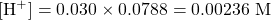With this [H+], then [OH] can be calculated as follows: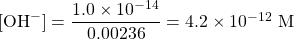This is about 30 times higher than would be expected for a strong acid of the same concentration.

Test Yourself
A 0.0222 M solution of pyridine (C5H5N) is 0.44% ionized into pyridinium ions (C5H5NH+) and OH ions. What are [OH] and [H+] for this solution?

[OH] = 9.77 × 10−5 M; [H+] = 1.02 × 10−10 M

Key Takeaways

• In any aqueous solution, the product of [H+] and [OH] equals 1.0 × 10−14.

Exercises

# Questions

1. Does [H+] remain constant in all aqueous solutions? Why or why not?
2. Does [OH] remain constant in all aqueous solutions? Why or why not?
3. What is the relationship between [H+] and Kw? Write a mathematical expression that relates them.
4. What is the relationship between [OH] and Kw? Write a mathematical expression that relates them.
5. Write the chemical equation for the autoionization of water and label the conjugate acid-base pairs.
6. Write the reverse of the reaction for the autoionization of water. It is still an acid-base reaction? If so, label the acid and base.
7. For a given aqueous solution, if [H+] = 1.0 × 10−3 M, what is [OH]?
8. For a given aqueous solution, if [H+] = 1.0 × 10−9 M, what is [OH]?
9. For a given aqueous solution, if [H+] = 7.92 × 10−5 M, what is [OH]?
10. For a given aqueous solution, if [H+] = 2.07 × 10−11 M, what is [H+]?
11. For a given aqueous solution, if [OH] = 1.0 × 10−5 M, what is [H+]?
12. For a given aqueous solution, if [OH] = 1.0 × 10−12 M, what is [H+]?
13. For a given aqueous solution, if [OH] = 3.77 × 10−4 M, what is [H+]?
14. For a given aqueous solution, if [OH] = 7.11 × 10−10 M, what is [H+]?
15. What are [H+] and [OH] in a 0.344 M solution of HNO3?
16. What are [H+] and [OH] in a 2.86 M solution of HBr?
17. What are [H+] and [OH] in a 0.00338 M solution of KOH?
18. What are [H+] and [OH] in a 6.02 × 10−4 M solution of Ca(OH)2?
19. If HNO2 is dissociated only to an extent of 0.445%, what are [H+] and [OH] in a 0.307 M solution of HNO2?
20. If (C2H5)2NH is dissociated only to an extent of 0.077%, what are [H+] and [OH] in a 0.0955 M solution of (C2H5)2NH?

1. [H+] varies with the amount of acid or base in a solution.
1. [H+] = Kw[OH]
1. H2O + H2O → H3O+ + OH; H2O/H3O+ and H2O/OH
1. 1.0 × 10−11 M
1. 1.26 × 10−10 M
1. 1.0 × 10−9 M
1. 2.65 × 10−11 M
1. [H+] = 0.344 M; [OH] = 2.91 × 10−14 M
1. [OH] = 0.00338 M; [H+] = 2.96 × 10−12 M
1. [H+] = 0.00137 M; [OH] = 7.32 × 10−12 M